# Season 1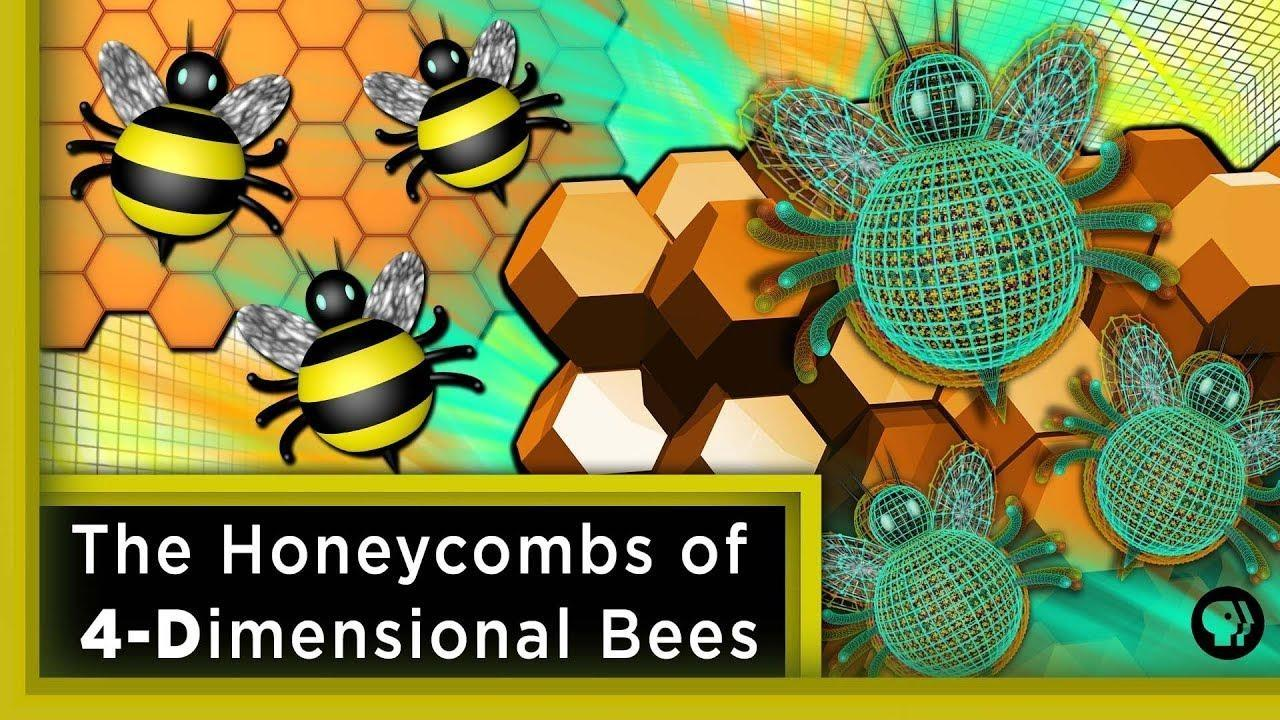Infinite Series

The Honeycombs of 4-Dimensional Bees ft. Joe Hanson

Why is there a hexagonal structure in honeycombs? Why not squares?

### The Honeycombs of 4-Dimensional Bees ft. Joe Hanson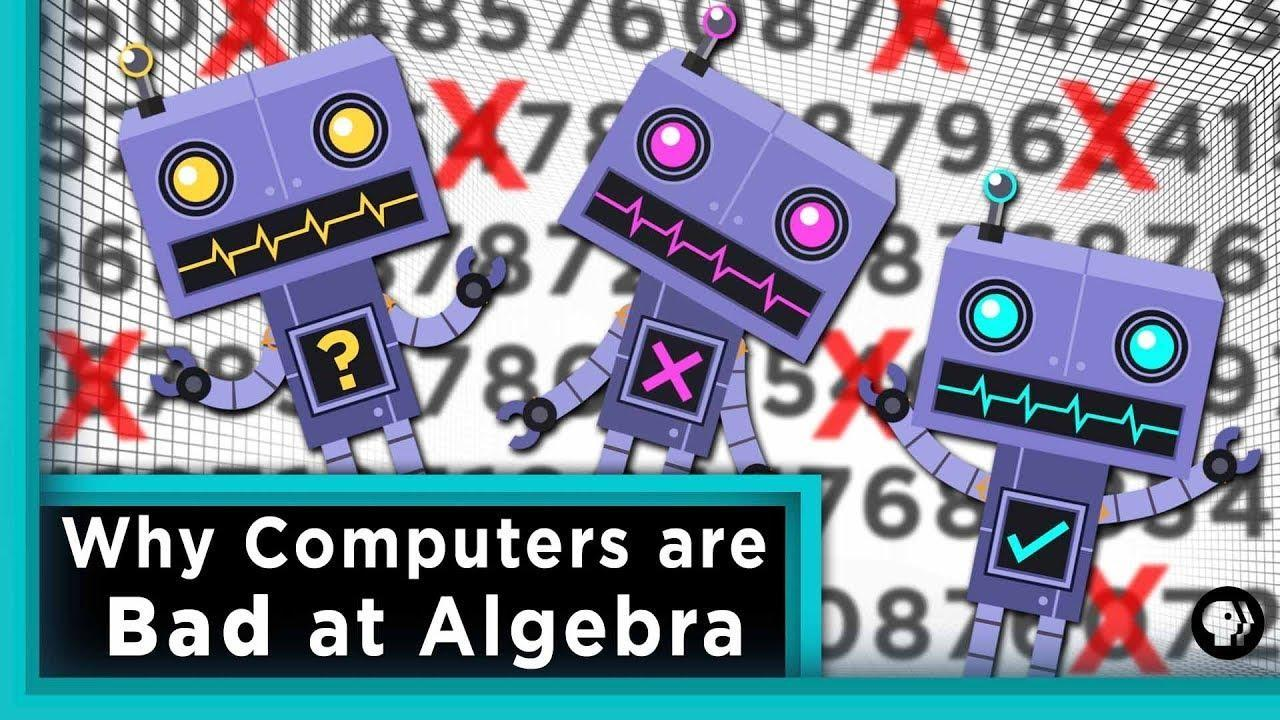Infinite Series

Why Computers are Bad at Algebra

The answer lies in the weirdness of floating-point numbers and the computer's perception..

### Why Computers are Bad at AlgebraInfinite Series

Making Probability Mathematical

What happened when a gambler asked for help from a mathematician?

### Making Probability Mathematical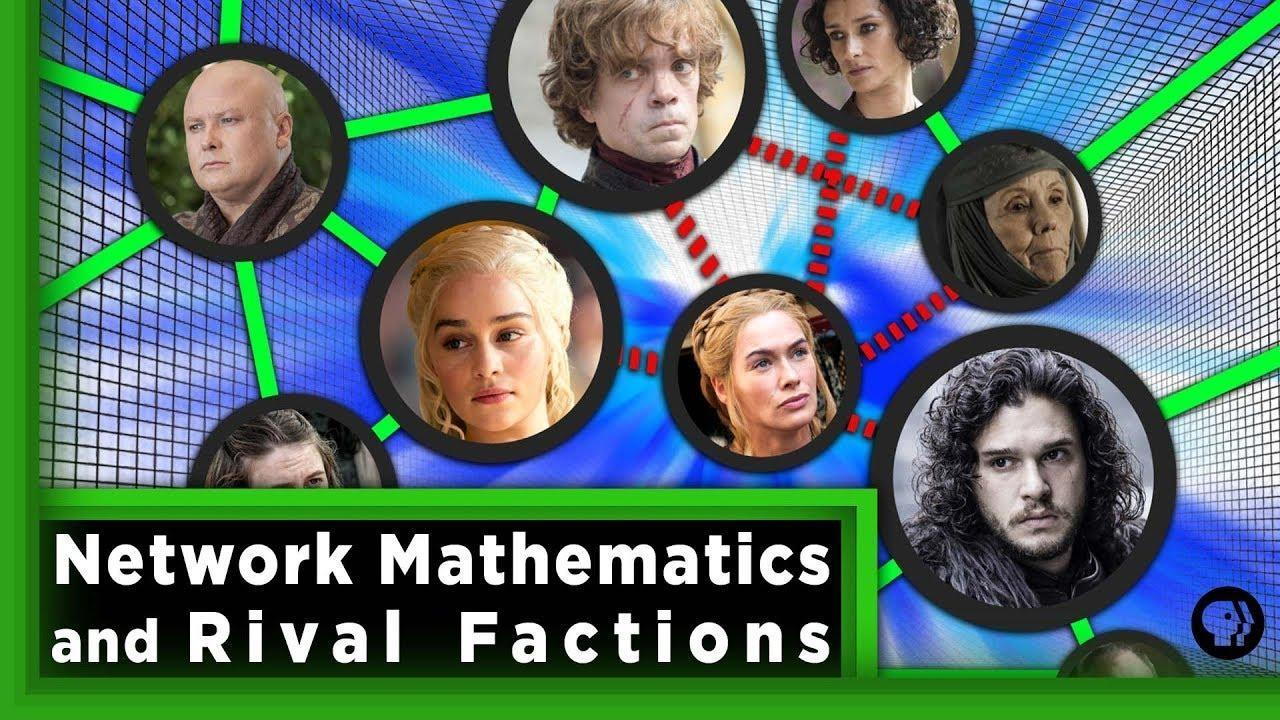Infinite Series

Network Mathematics and Rival Factions | Infinite Series

The theory of social networks allows us to mathematically model and analyze..

### Network Mathematics and Rival Factions | Infinite SeriesInfinite Series

Arrow's Impossibility Theorem

The bizarre Arrow’s Impossibility Theorem, or Arrow’s Paradox.

### Arrow's Impossibility TheoremInfinite Series

Voting Systems and the Condorcet Paradox

What is the best voting system?

### Voting Systems and the Condorcet ParadoxInfinite Series

Dissecting Hypercubes with Pascal's Triangle

What does the inside of a tesseract look like? Pascal’s Triangle can tell us.

### Dissecting Hypercubes with Pascal's TriangleInfinite Series

Topology Riddles | Infinite Series

Can you turn your pants inside out without taking your feet off the ground?

### Topology Riddles | Infinite SeriesInfinite Series

Building an Infinite Bridge

Using the harmonic series we can build an infinitely long bridge.

### Building an Infinite BridgeInfinite Series

Hacking at Quantum Speed with Shor's Algorithm

Classical computers struggle to crack modern encryption.

### Hacking at Quantum Speed with Shor's AlgorithmInfinite Series

How to Break Cryptography

Only 4 steps stand between you and the secrets hidden behind RSA cryptography.

### How to Break Cryptography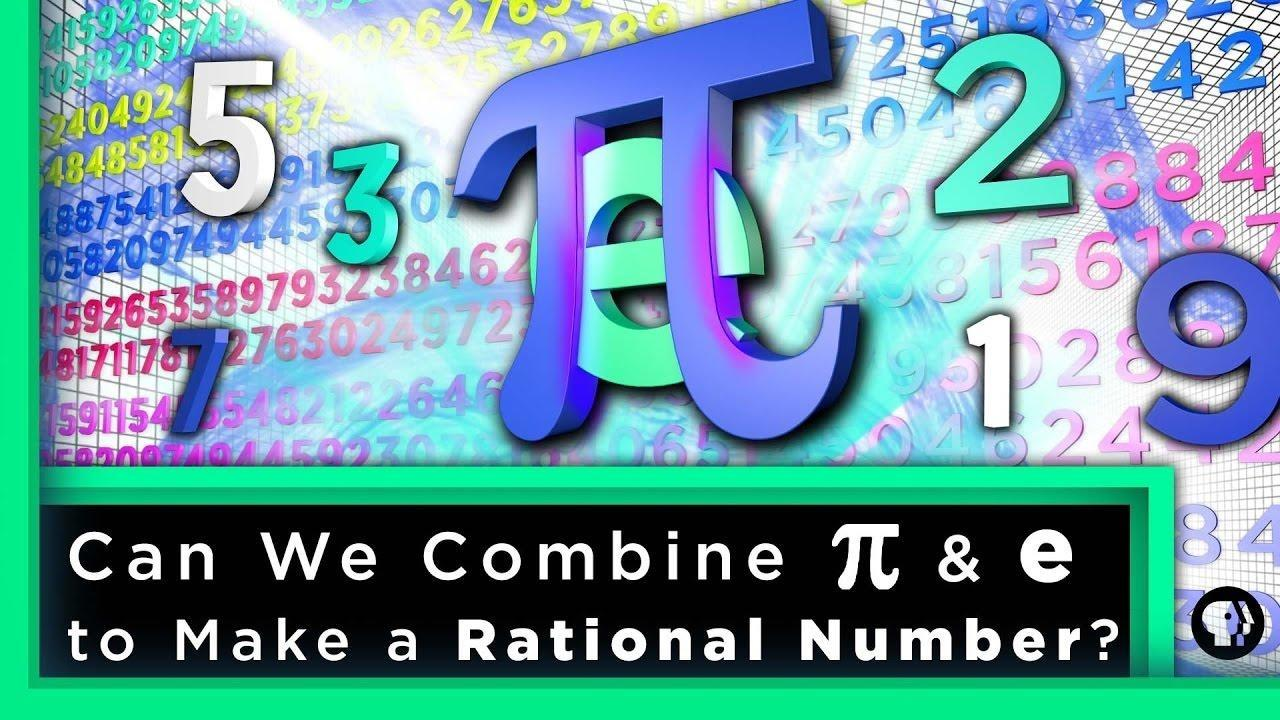Infinite Series

Can We Combine pi & e to Make a Rational Number?

Can you produce a rational number by exchanging infinitely many digits of pi and e?

### Can We Combine pi & e to Make a Rational Number?Infinite Series

Solving the Wolverine Problem with Graph Coloring

At one time, Wolverine served on four different superhero teams. How did he do it?

### Solving the Wolverine Problem with Graph Coloring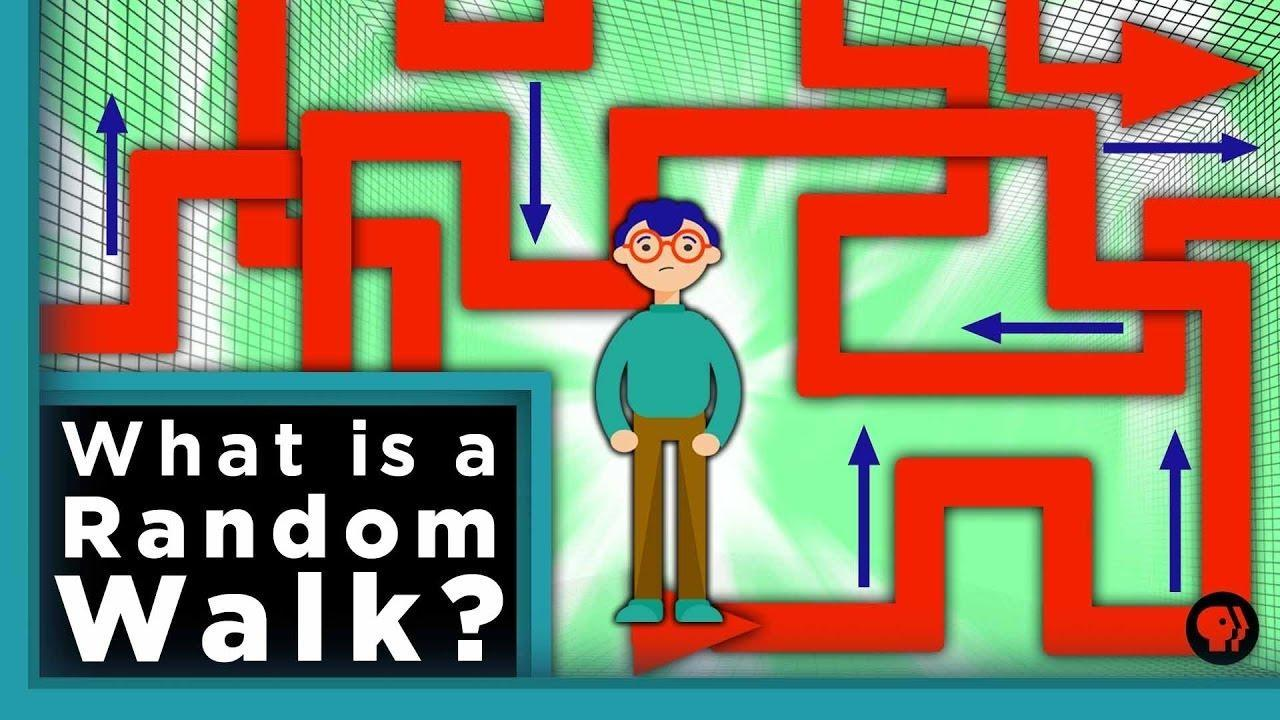Infinite Series

What is a Random Walk?

To understand finance, search algorithms and even evolution you need to understand Random

### What is a Random Walk?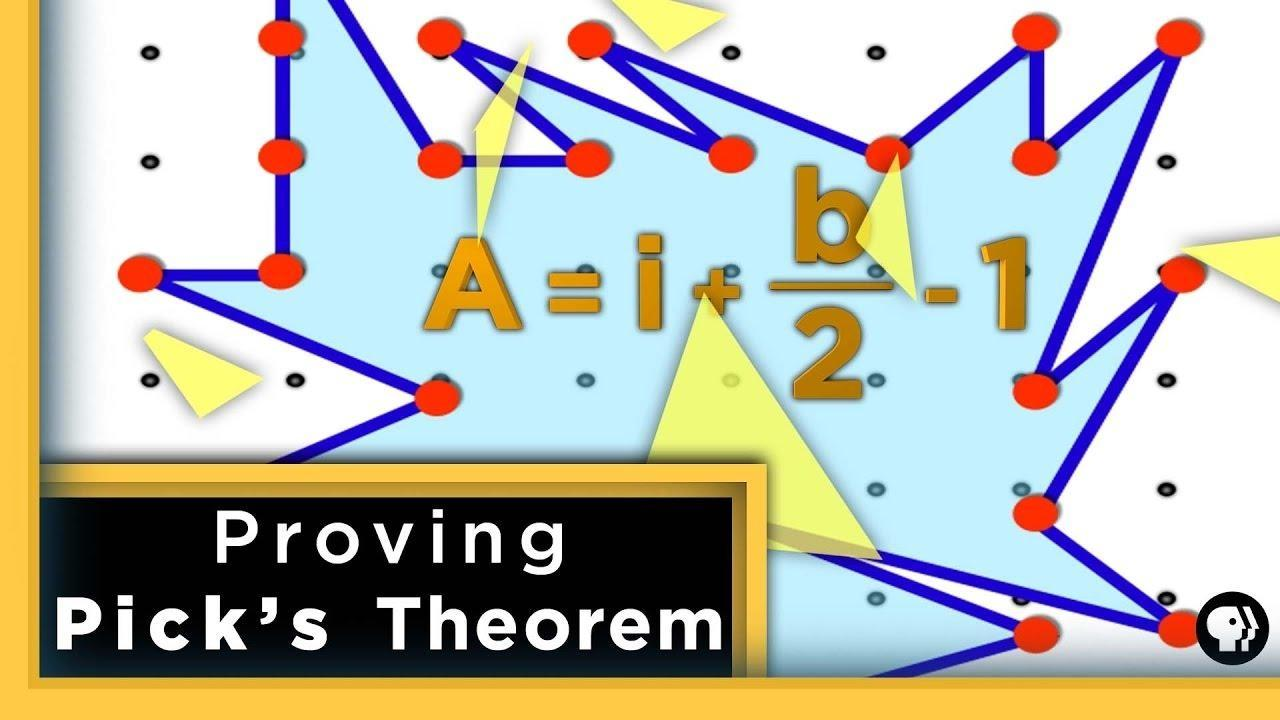Infinite Series

Proving Pick's Theorem

What is Pick's Theorem and how can we prove it?

### Proving Pick's Theorem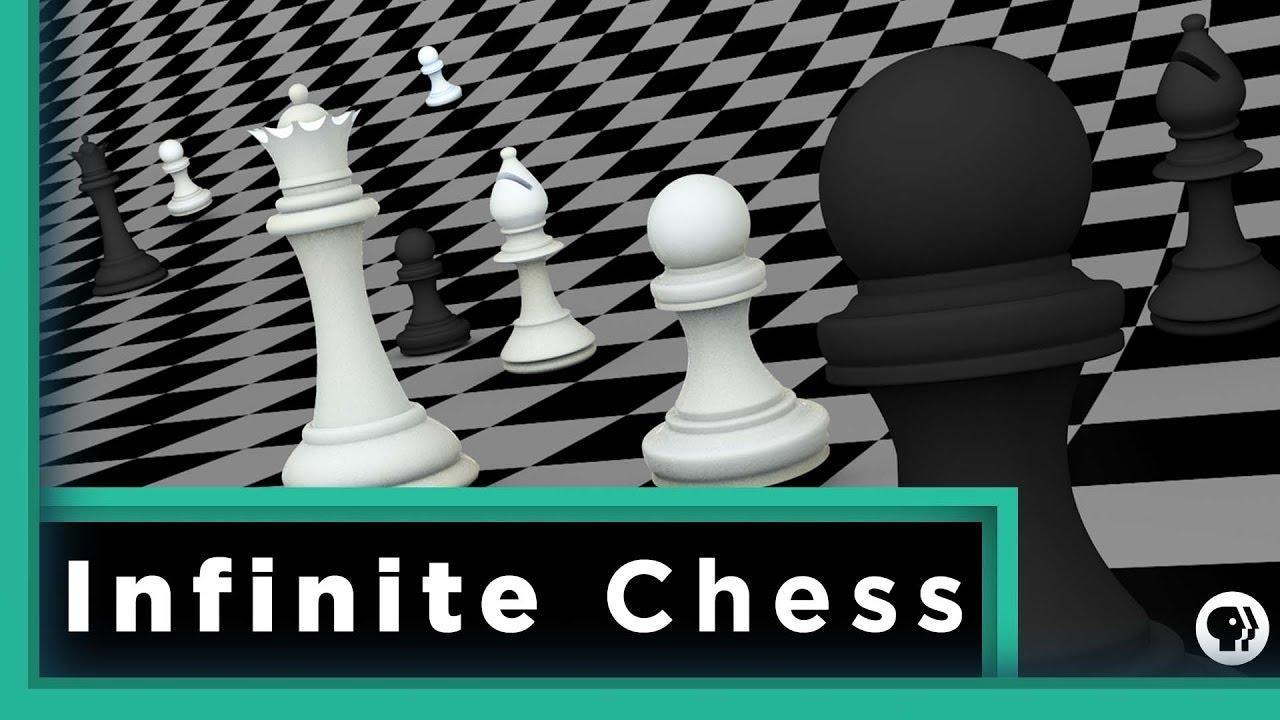Infinite Series

Infinite Chess

How long will it take to win a game of chess on an infinite chessboard?

### Infinite ChessInfinite Series

Splitting Rent with Triangles

You can find out how to fairly divide rent between three different people even when you do

### Splitting Rent with TrianglesInfinite Series

The Mathematics of Quantum Computers

What is the math behind quantum computers? And why are quantum computers so amazing?

### The Mathematics of Quantum Computers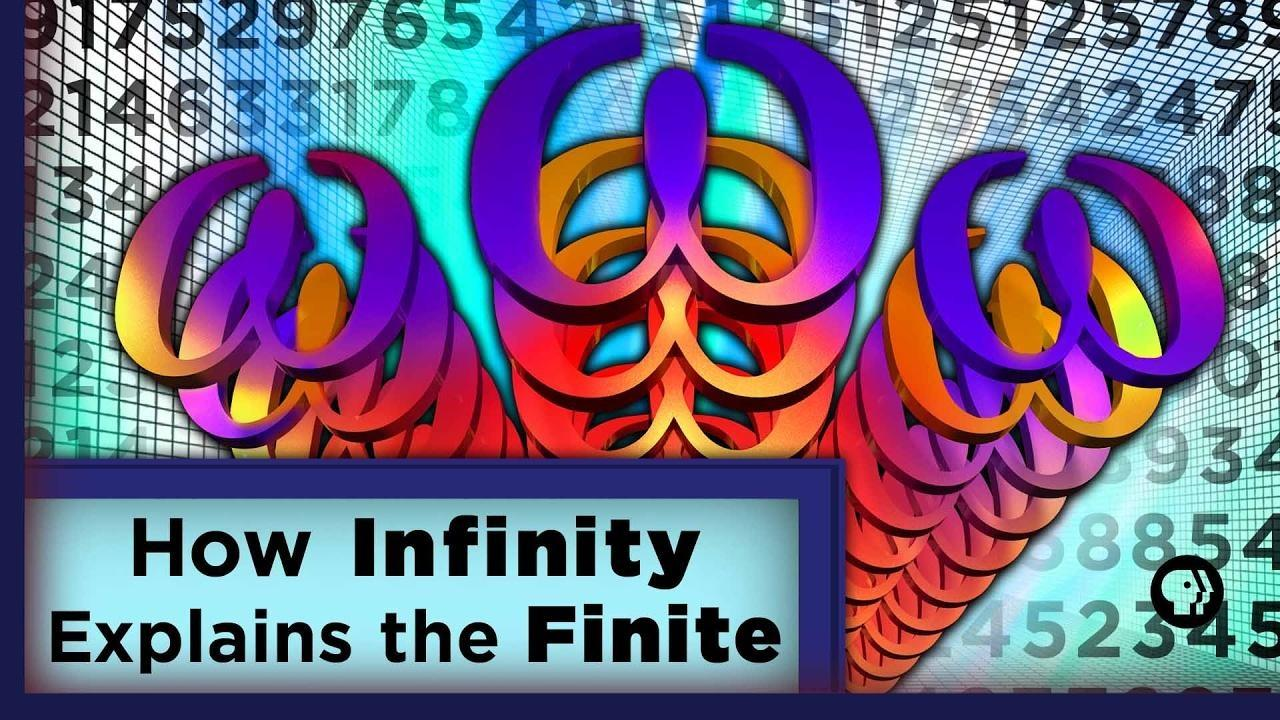Infinite Series

How Infinity Explains the Finite

Peano arithmetic proves many theories in mathematics but does have its limits.

### How Infinity Explains the FiniteInfinite Series

Kill the Mathematical Hydra

How do you defeat a creature that grows two heads for every one head you chop off?

### Kill the Mathematical HydraInfinite Series

Singularities Explained

Mathematician Kelsey Houston-Edwards explains exactly what singularities are.

### Singularities Explained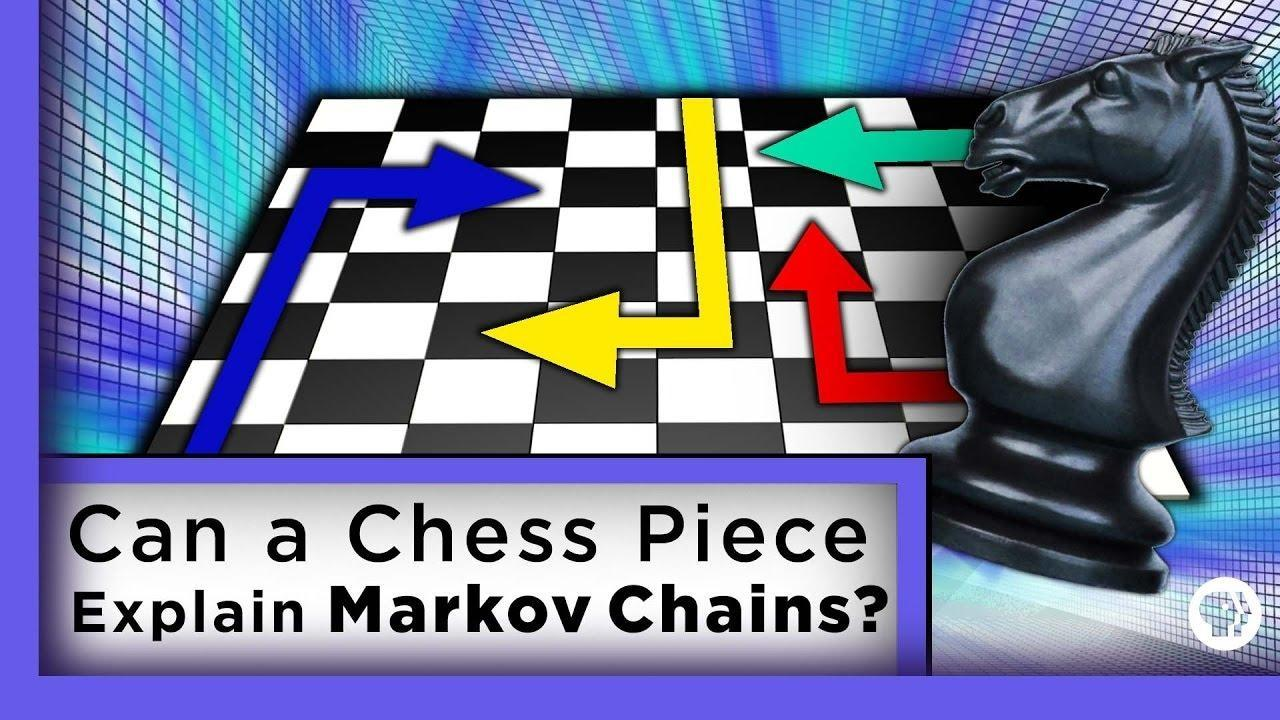Infinite Series

Can a Chess Piece Explain Markov Chains?

In this episode probability mathematics and chess collide.

### Can a Chess Piece Explain Markov Chains?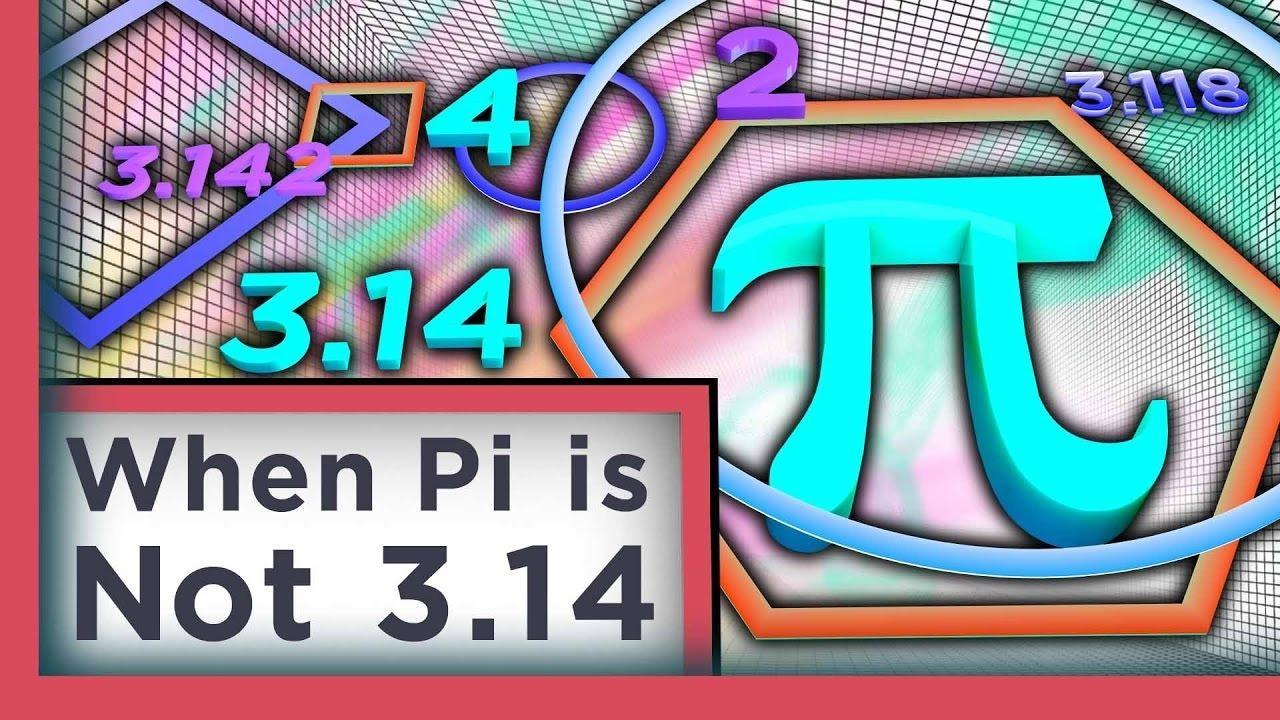Infinite Series

When Pi is Not 3.14

You’ve always been told that pi is 3.14. This is true, but this number is based on...

### When Pi is Not 3.14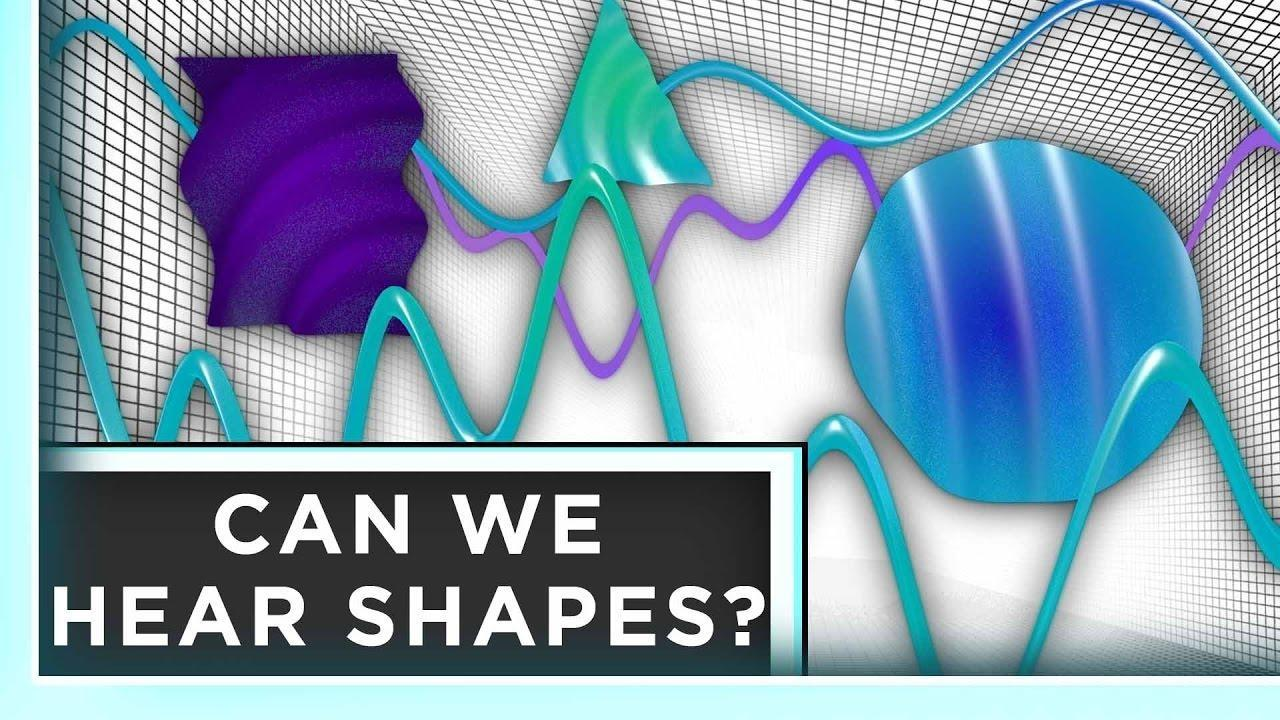Infinite Series

Can We Hear Shapes?

Mathematician Mark Kac asked the question “Can we hear the shape of a drum?”